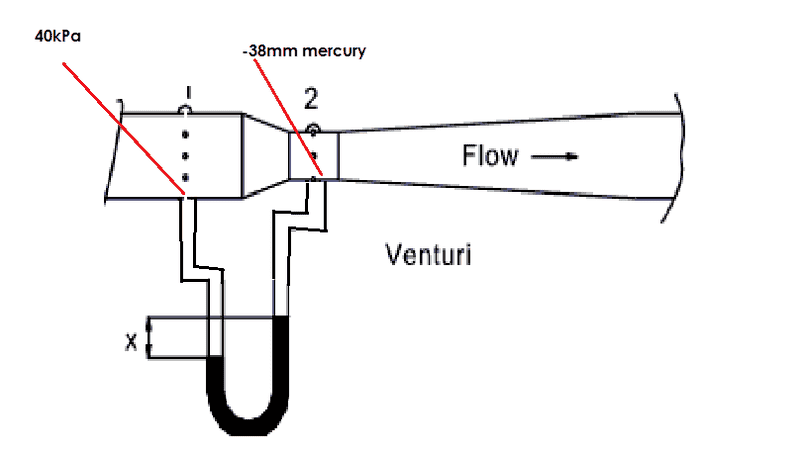# Fluid Mechanics: Pressure in Fluids

• Lukas_RSA

## Homework Statement

The gauge pressure at the inlet of a horizontal venturi meter is 40kPa. The vacuum at the throat is 38 mm mercury. If a differential U-tube manometer containing mercury is the U-tube and the oil in the connecting tubes is connected to the venturi, what will the difference in the mercury levels be? the fluid in the venturi meter is oil with a relative density of 0.8. Answer [0,359m]

## The Attempt at a Solution

I have no idea how to start with this problem, i am assuming to use the following formulas but i don't understand them,

P1 - P1 / (p_oil)(g) = (S_m - 1) h_m

but i don't know how to get the answer, please assist me with this, i am going to youtube now to look for some tutorials on the u-tube manometer, you can also refer me to a great tutorial :-)What do you do when you want to compare two quantities with the same dimension but expressed in different units ?

hi BvU

thanks for you response sorry for taking so long to respond but i am busy studying so my time on the pc is a bit limited :-)

i am trying to convert the 38mm of mercury to a pressure so that i can then use the conservation method, put one side equal to the other side, then try to determine the height of the difference in mercury, but when i use P2=pgh and substitute the 38 mm in h i don't get the answer i am looking for, is this approach even the right approach?
after the P2=pgh

i put P1 = P2+ p(mercury)gh(x value)

40 000 = (-5068) + 133416x
40 000 + 5068 / 133416 = x
x = 0.3378m

the textbook says the answer should be 0.359m, is my logic wrong?

i hope to hear from you soon.

regards
Lukas van Rooyen

Hello Lukas,

Several remarks:
Apparently you do everything in terms of gauge pressure. I'm a physicist and find it infinitely safer to work with actual (absolute) pressures. Never mind.

Your ##-##5068 is ##\rho\,g\,h## (but 38 mm * 13690 kg/m3 * 9.81 m/s2 = 5100 kg m/s2.!?) .  my mistake -- ##\rho## is smaller

What they mean when they say the vacuum is 38 mm is a mystery to me. Perhaps they indeed mean the gauge pressure is ##-##38 mm (you'll have to get that out of the context of your book I suppose), in which case I can understand your calculation (but I get 335.8 mm).
But that's assuming the whole story
If a differential U-tube manometer containing mercury is the U-tube and the oil in the connecting tubes is connected to the venturi, what will the difference in the mercury levels be? the fluid in the venturi meter is oil with a relative density of 0.8.
doesn't change a thing. But it does ! -- if you make one assumption about this venturi meter: that it's used for a gas with negligible density.

Last edited: